# 机器学习【二】广义线性模型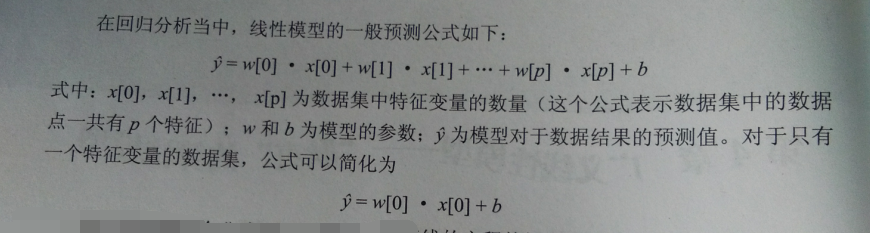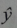读作“ y hat ”  ，代表y的估计值

import numpy as np
import matplotlib.pyplot as plt
#令x为-5，5之间，元素数为100的等差数列
x = np.linspace(-5,5,100)
#输入直线方程
y = 0.5*x+3
plt.plot(x,y,c='orange')
plt.title('Straight Line')
plt.show()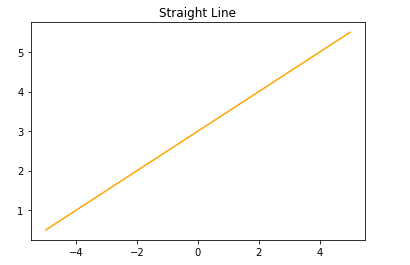1.由（1，3），（4，5）两点画直线

#导入线性回归模型
from sklearn.linear_model import LinearRegression
#输入二点横坐标
X = [,]
#输入二点纵坐标
y = [3,5]
#用线性模型拟合这两个点
lr = LinearRegression().fit(X,y)
#画出两个点和直线的图形
z = np.linspace(0,5,20)
plt.scatter(X,y,s=80)
plt.plot(z, lr.predict(z.reshape(-1,1)),c='k')
plt.title('Straight Line')
plt.show()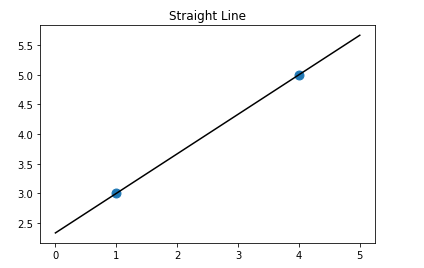print('斜率：',lr.coef_)
print('截距：',lr.intercept_)

斜率： 0.6666666666666664
截距： 2.333333333333334

2.新加（3，3）点，画直线：

#导入线性回归模型
from sklearn.linear_model import LinearRegression
#输入二点横坐标
X = [,,]
#输入二点纵坐标
y = [3,5,3]
#用线性模型拟合这三个点
lr = LinearRegression().fit(X,y)
#画出两个点和直线的图形
z = np.linspace(0,5,20)
plt.scatter(X,y,s=80)
plt.plot(z, lr.predict(z.reshape(-1,1)),c='k')
plt.title('Straight Line')
plt.show()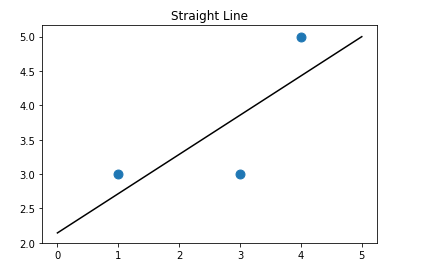print('斜率：',lr.coef_)

print('截距：',lr.intercept_)

斜率： 0.5714285714285713

线性回归模型的原理：

3.利用sklearn生成的make_regression数据集为例：

from sklearn.datasets import make_regression
#生成用于回归分析的数据集
X,y = make_regression(n_samples=50,n_features=1,n_informative=1,noise=50,random_state=1)
#使用线性模型对数据进行拟合
reg = LinearRegression()
reg.fit(X,y)
#z是我们生成的等差数列，用来画出线性模型的图形
z = np.linspace(-3,3,200).reshape(-1,1)
plt.scatter(X,y,c='b',s=60)
plt.plot(z,reg.predict(z),c='k')
plt.title('Linear Regression')
plt.show()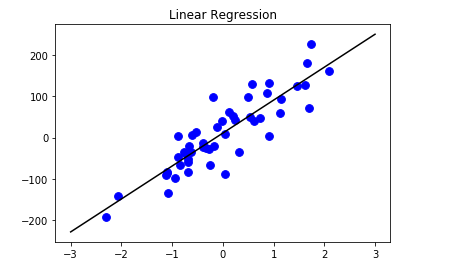print('斜率：',reg.coef_)print('截距：',reg.intercept_)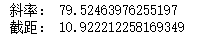可能注意到 coef_ 和 intercept_ 这两个属性以 '_' 结尾

线性模型特点

以上使用的都是特征数只有1个的数据集

1.线性回归的基本原理：

#使用make_regression函数，生成一个样本数量为100，特征数量为2的数据集，并利用 train_test_split 函数将数据集分割成训练集和测试集，再用线性回归模型计算出w、b值

#导入数据集拆分工具
from sklearn.model_selection import train_test_split
from sklearn.linear_model import LinearRegression
X,y = make_regression(n_samples=100,n_features=2,n_informative=2,random_state=38)
X_train,X_test,y_train,y_test = train_test_split(X,y,random_state=8)
lr = LinearRegression().fit(X_train,y_train)
print('斜率w:',lr.coef_[:])
print('截距b:',lr.intercept_)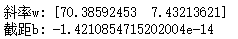intercept_ 属性一直是是一个浮点数，而coef_ 属性则是一个Numpy的数组【其中每个特征对应数据中的一个数值】

#由于使用的make_regression 生成的数据集中数据点有2个特征，所以lr.coef_ 是一个二维数组

y = 73.3859 * X1 + 7.4321 * X2 - 1.42e-14

2.线性回归的性能表现

print(lr.score(X_train,y_train))
print(lr.score(X_test,y_test))

1.0
1.0

没有向数据集中加入noise,自然满分

3.真实的数据集：【特征多，noise多】

#载入糖尿病数据集
#将数据集拆分为训练集和测试集
X_train,X_test,y_train,y_test = train_test_split(X,y,random_state=8)
#使用线性模型拟合
lr = LinearRegression().fit(X_train,y_train)
#得分
print(lr.score(X_train,y_train))
print(lr.score(X_test,y_test))

0.5303814759709331
0.45934404966916426

#在训练集和测试集上得分的巨大差异——> 出现过拟合问题，所以应该找到一个模型，是我们能够控制模型的复杂度——> 岭回归

【实际上，是一种改良的最小二乘法】

【一种能够避免过拟合的线性模型】

1.原理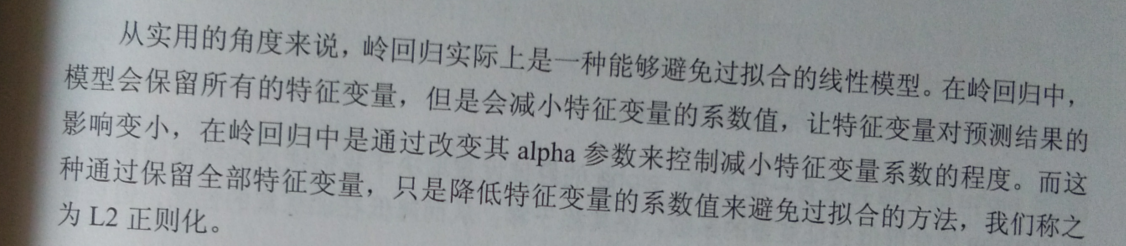#岭回归在sklearn中通过linear_model.Ridge 函数来调用

#导入岭回归
from sklearn.linear_model import Ridge
#使用岭回归对数据拟合
rd = Ridge().fit(X_train,y_train)
#得分
print(rd.score(X_train,y_train))
print(rd.score(X_test,y_test))

0.4326376676137663
0.43252177690681864

#复杂度越低的模型。在训练集上表现的越差，但是其泛化的能力更好，如果我们在意的是模型在泛化上的表现，就应该选择岭回归

2.参数调节

【岭回归是在模型的简单性（使系数趋近于0）和它在训练集上的性能之间取得平衡的一种模型】

【上方例子使用默认参数alpha = 1】#导入岭回归
from sklearn.linear_model import Ridge
#使用岭回归对数据拟合，修改alpha参数为10
rd10 = Ridge(alpha=10).fit(X_train,y_train)
#得分
print(rd10.score(X_train,y_train))
print(rd10.score(X_test,y_test))

0.15119962367011153
0.16202013428866247

#测试得分>训练得分，说明如果我们的模型出现了过拟合现象，可以提高alpha的值来降低过拟合的程度

#导入岭回归
from sklearn.linear_model import Ridge
#使用岭回归对数据拟合，修改alpha参数为0.1
rd1 = Ridge(alpha=0.1).fit(X_train,y_train)
#得分
print(rd1.score(X_train,y_train))
print(rd1.score(X_test,y_test))

0.5215646055241339
0.4734019500945309

【可以尝试降低alpha的值，改善模型的泛化表现 { 测试得分增大 } 】

【较高的 alpha 代表模型的限制更严格，所以高 alpha 下，coef_数值更大】

#导入岭回归
from sklearn.linear_model import Ridge
#使用岭回归对数据拟合
rd = Ridge(alpha=1).fit(X_train,y_train)
rd10 = Ridge(alpha=10).fit(X_train,y_train)
rd0 = Ridge(alpha=0.1).fit(X_train,y_train)
#绘制alpha=1时的模型参数
plt.plot(rd.coef_, 's',label='Ridge alpha=1')
plt.plot(rd10.coef_, '^',label='Ridge alpha=10')
plt.plot(rd0.coef_, 'v',label='Ridge alpha=0.1')
#绘制线性回归的系数作对比
plt.plot(lr.coef_, 'o',label='linear regression')
plt.xlabel('coefficient index')
plt.ylabel('coefficient magitude')
plt.hlines(0,0,len(lr.coef_))
plt.legend()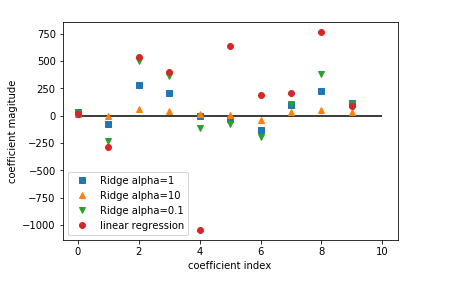X=0 显示第一个特征变量系数，X=1显示的是第二个特征变量的系数 。。。。。

alpha=0.1时，系数更大，甚至大部分与线性回归的点重合

而线性模型没经过任何正则处理，所以特征变量系数会非常大

另一个理解正则化对模型影响的方法——取一个固定的alpha，改变训练集的数据量

from sklearn.model_selection import learning_curve,KFold
#定制一个学习曲线的函数
def plot_learning_curve(est,X,y):
#将数据进行20次拆分用来对模型进行评分
training_set_size,train_scores,test_scores = learning_curve(est,X,y,train_sizes=np.linspace(.1,1,20),cv=KFold(20,shuffle=True,random_state=1))
estimator_name = est.__class__.__name__
line = plt.plot(training_set_size,train_scores.mean(axis=1),'--',label='training '+estimator_name)
plt.plot(training_set_size, train_scores.mean(axis=1),'-',label='test' + estimator_name,c=line.get_color())
plt.xlabel('Training set size')
plt.ylabel('Score')
plt.ylim(0,1.1)
plot_learning_curve(Ridge(alpha=1),X,y)
plot_learning_curve(LinearRegression(),X,y)
plt.legend(loc=(0,1.05),ncol=2,fontsize=11)

【书上的总共会出现4条曲线，但代码一样，原因未知，所以选取书上例图】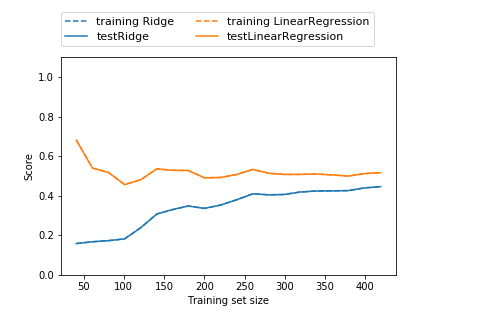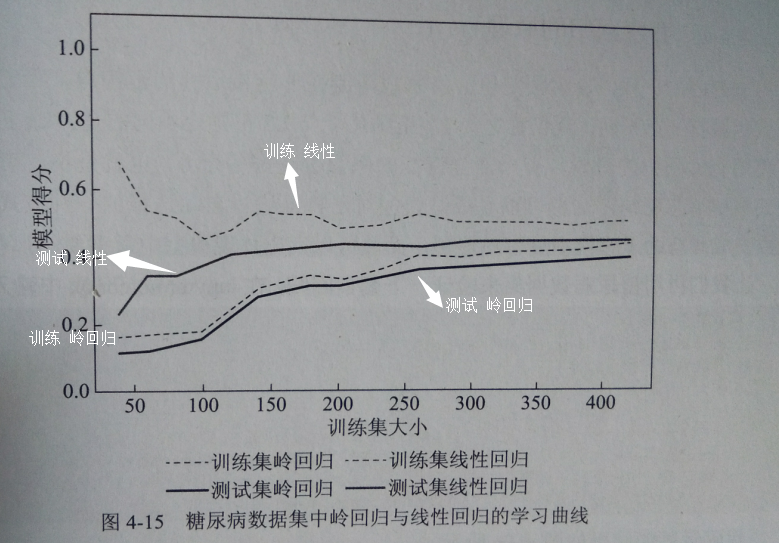1.无论岭回归还是线性回归，训练集>测试集

2.岭回归是经过正则化的模型，所以，整个图像中，训练集要比线性回归的低

3.岭回归在 训练集 和 测试集 的得分差异低【尤其在数据子集比较小的情况】

4.数据量小于50，线性回归几乎让机器学不到任何东西

5.随着数据规模增大，线性回归的得分赶上了岭回归的得分——>【足够多的数据，正则化显得不重要，两模型表现差不多】

#随着数据量的增大，线性回归在训练集的得分下降——> 随着数据增加，线性模型越不容易产生过拟合现象 【即越难记住数据】

1.原理

from sklearn.linear_model import Lasso
#使用套索回归拟合数据
lasso = Lasso().fit(X_train,y_train)
#得分
print(lasso.score(X_train,y_train))
print(lasso.score(X_test,y_test))

print(np.sum(lasso.coef_ != 0))  #使用的特征数

0.36242428249291325
0.365618589621283

2.参数调节

降低alpha的值 ——> 降低欠拟合的程度【同时还需要增大最大迭代次数】

#增大模型迭代次数的默认设置
#否则模型会提示我们增加最大迭代次数
lasso01 = Lasso(alpha=0.1,max_iter=100000).fit(X_train,y_train)
#得分 & 特征数
print(lasso01.score(X_train,y_train))
print(lasso01.score(X_test,y_test))
print(np.sum(lasso01.coef_ != 0))

0.519480608218357
0.47994757514558173
7

#把alpha 设置为0.0001
lasso000= Lasso(alpha=0.0001,max_iter=100000).fit(X_train,y_train)
#得分 & 特征数
print(lasso000.score(X_train,y_train))
print(lasso000.score(X_test,y_test))
print(np.sum(lasso000.coef_ != 0))

0.5303811330981303
0.4594509683706017
10

#使用全部的特征，而且测试集得分略低于alpha=0.1的情况——> 降低alpha 倾向让模型出现过拟合现象

比较不同alpha 值的套索回归和岭回归进行系数对比：

#绘制alpha 值等于1时的模型系数
plt.plot(lasso.coef_ ,'s',label='Lasso alpha=1')
#绘制alpha 值等于0.1时的模型系数
plt.plot(lasso01.coef_, '^',label='Lasso alpha=0.1')
#绘制alpha 值等于0.001时的模型系数
plt.plot(lasso000.coef_, 'v',label='Lasso alpha=0.0001')
#绘制alpha=0.1 的岭回归模型系数作为对比
plt.plot(ridge01.coef_,'o',label='Ridge alpha=0.1')
plt.legend(ncol=2,loc=(0,1.05))
plt.ylim(-1000,800)
plt.xlabel('Coefficient index')
plt.ylabel('Coefficient magnitude')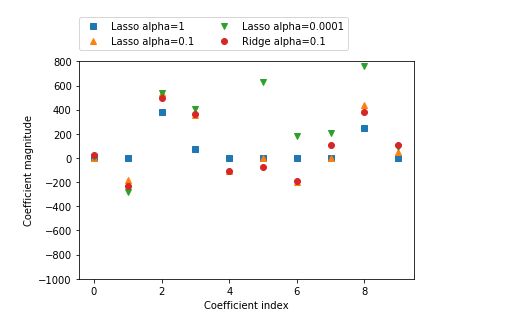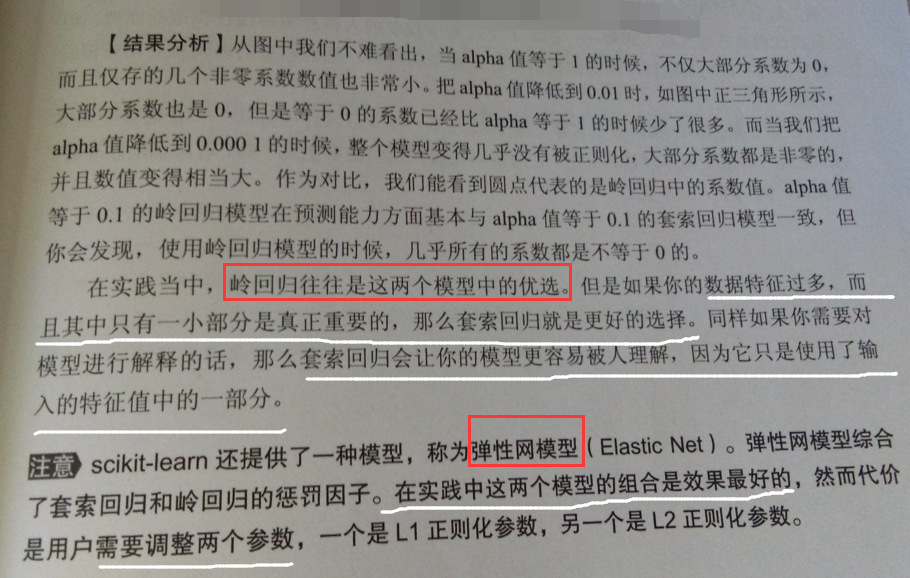posted @ 2019-04-17 15:25  远征i  阅读(352)  评论(0编辑  收藏